Of Torus and Turks-Head Knots: A Polar Trigonometric Modeling By Skip Pennock 3333 N. Charles St. Appt. 600 Baltimore, MD 21218-3213, USA Skippyknotty@aol.com   This article concerns itself with graphical solutions from an artists/ craftspersons/ knot tyers perspective. The knots depicted may actually be tied utilizing the diagrams contained herein, although a good many of the knots may also be tied in the hand, as a sailor/ boater would say, because these knots are kept relatively simple for illustrating purposes. Nonetheless, for knots that are more complex, some form of a graphical solution is very often resorted to. You are very likely to know more about mathematics than I will ever know. I apologize, taking into account your knowledge. In all probability this work has been done before my attempts. It is simply that I do not know of these other attempts, and have never seen these graphs before, elsewhere. The mathematics presented herein, I should say, consists of many anecdotal cases, for, not being a true mathematician, I do not know how to generalize them into a formal proof. Figure 1 shows an additive model applied for the centerlines of two very simple Turks-Head knots. The equations are also very simple. If the radius R = a constant (in this case, 2 units), then we have the graph of a circle. If an appropriate wave is added to the circle, then we will have a wavy, braided graph with the right number of Bights and the right number of Leads.Figure 1 Top: R = 2 + cos( 3q / 2 ) ( 0 < q < 2 × 360 = 720 ) Bottom: R = 2 + cos( 4q / 3 ) ( 0 < q < 3 × 360 = 1080 ) In these cases, a Cosine wave was chosen, because, at its start where q, q = 0o, (or q = 0 Radians), there will be a maximum, and an axis (or orthogonal plane) of symmetry. q ranges between 0 (0 radians), and the Number of Leads × 360 degrees (#Leads × 2p radians). The Amplitude of the Cosine wave was set to = an implicit 1 unit, for reasons of simplification. Some definitions concerning Turks-Head Knots, Bights, and Leads (pronounced leeds) are in order here. To be respectful, a word about the nomenclature Turks-Head knots, for were I of Turkish lineage, I might be offended by the term: The name first appeared in the literature in Darcy Levers 1808 publication The Sheet Anchor , according to Clifford W. Ashley, in his comprehensive and unequaled to date publication, The Ashley Book of Knots. Ashley goes on, saying Their resemblance to turbans was presumably the reason for the name, and There are no knots with a wider field of usefulness. The family of Single Piece, or Common, or Running Turks-Head knots, as they are now known almost universally, is one of the most attractive series of knots ever devised or discovered. They are quite ancient, with representations of them being carved into columns found in Egyptian Pharaonic tombs, for example. They are usually cylindrical in form, but they can be tied in decorative and practical woven flat knotting form. Besides consisting of single lengths of cordage, once they are finished, they are Alternating knots with a regular over/under, sometimes termed Basket weave, pattern. A Bight is defined as a scallop or curve at the rim of the knot where the cord changes direction. Given a Common, Running Turks-Head knot, there is only one number of Bights. For a flat knot, if you count, the number is the same around the inside hole as around the outside rim of the disk formed by the knot. For a cylindrical knot, the number at the top of the cylinder always equals the number at the bottom. A Lead is defined for a Turks-Head knot as a single path or revolution of the cord around the band, or if a flat knot, around the hole in the center of the disk, while discounting the extra parallel threading or following of the original knot when an extra Ply or two is put into the knot. (Sailors call putting an extra ply or two into a knot, doubling and tripling or, doubling twice. A little confusing terminology and tradition.) Given a Turks-Head knot, there is only one number of Leads. Figure 2 shows a Turks-Head knot tying diagram, which was created for use with copper twist wire. For knots which are tied of softer material, such as most cordage, this knot may be made without resort to nails, pins, or diagram, and may easily be tied in the hand.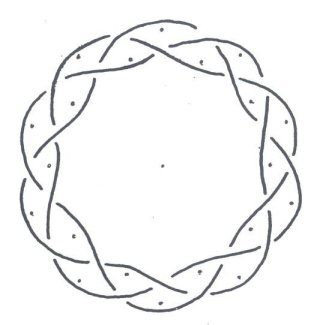Figure 2 A Knot Tying Diagram for a 10-Bight, 3-Lead Turks-Head Bracelet Knot. The dots show locations of pins/nails utilized as guideposts. Depending upon how one views graphical representation, Figure 3 either shows a doubled or tripled centerline diagram. The cord could be viewed as the space between two lines. This would be the most common choice for a sailor, and results in a doubled knot. Or, alternatively, the cord could be viewed as being made up by the lines themselves, which would probably be the favored representation by a knot theorist, and would result in a tripled knot, or in other words a knot with three plies. Something that is unresolved in either case in Figure 3, is exactly how the ends of the cord are to be finished off, which in practical terms usually means hiding them on the underside of the knot where they will not show from above, and seizing them into place. Figure 3 is actually three equations, expressed together as one, through the usage of braces. And just as in Figure 2, there are 10 Bights and 3 Leads.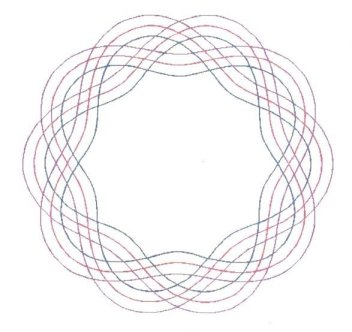Figure 3 R = ( 5.0, 5.5, 6.0 ) + cos( 10q / 3 ) ( 0 < q < 3 × 360 = 1080 ) Something that needs mentioning is that in all the models of Running Turks-Head knots in this article, and all known of, the number of Bights and the Number of Leads may have no common factor (or, divisor). The models explain this phenomenon. If they do, in the models, the factor will cancel out in both the numerator (#Bights) and the denominator (#Leads). In such a case the resulting modeled knot will have both fewer Bights and fewer Leads, and it may not form a braided knot at all, but rather, the Unknot. It should be apparent that the generalized model used thus far is: Radius = Rmid + (Amp × Cosine ( B × T / L )) Where: Rmid is a Midrange radius constant, which is greater than Amp; Amp is an amplitude constant, equal to 1/2 of the width of the braid; B is the number of Bights, a cardinal (counting) number; L is the number of Leads, a cardinal number, greater than 1; and, T is the independent variable q. Something which is surprising, is that very often, not only can Rmid be Added to the parenthetic Cosine function, but Rmid can be Raised to it. Figure 4 and Figure 5 demonstrate this. In these cases, Rmid is not actually a true midrange value.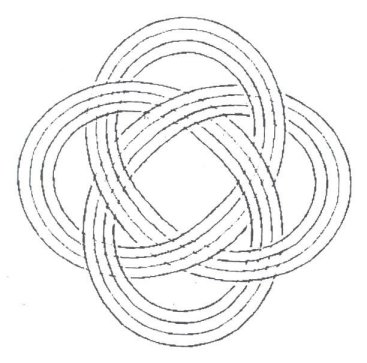Figure 4 This Drawing shows some of the beauty present in a tripled Turks-Head Knot, though depicted in black & white.Figure 5 R = ( 0.25, 0.50, 0.75, 1.00 ) + 2 (cos( 4q / 3) Figure 6 and Figure 7 show the versions of some of the first, additive models the author arrived upon while taking a Calculus I refresher class at Towson University. One day after class, the Professor asked, What are all those pretty little geometric drawings you make during class? I explained and she suggested I utilize Polar Coordinates directly, instead of trying to convert over from the more familiar Cartesian Coordinate System. Figure 8 is the most recent personal result for an 8-Bight, 5-Lead graph.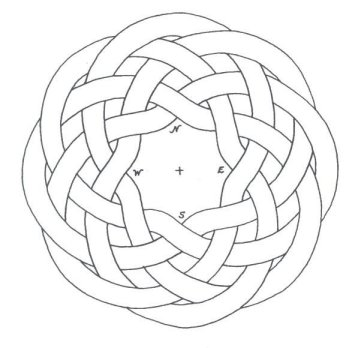Figure 6 A hand-made figure, with an outer set of curved line segments drawn parallel to the inner curved lines, plotted using polar graph paper, felt-tip pen, & calculator during early 1980s.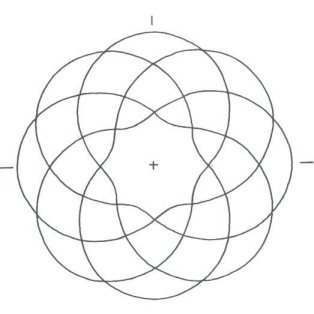Figure 7 R = 2 + cos ( 8q/ 5 ) ( 0 < q < 5 × 360 = 1800 ) Hand plotted and graphed.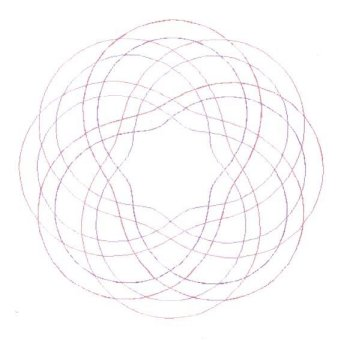Figure 8 R = ( 2.0, 2.4 ) + cos ( 8q/ 5 ) ( 0 < q < 5 × 360 = 1800 ) Computer plotted, utilizing a Graphing Calculator program package. The lines approach, but are not, strictly parallel. Figure 9 & Figure 10 are just for the fun of it.Figure 9 A Compass Rose. No, There is no North by Northwest, despite the movie title. All of the by names are among N, E, S, & W only. For example, there is a NW by N and also a NW by W. R = ( 2.0, 2.4 ) + cos ( 16q/ 5 )Figure 10 Having some fun, a Flower knot graph is designed. R = 0.75 and R = ( 2.0, 2.4 ) + cos ( 16q/ 3 ) Figure 11, as it says, works much like a clock. It explains just about all that the author knows about Music Theory, which is not a whole lot, but attention should be paid to the written part at the bottom of the figure. Here is an interesting application of a Knot to Music Theory. It works much like a clock. CYCLE of "FIFTHS" in Major Key SignaturesFigure 11 A Musical Pathway For the Cycle of Fifths, starting from the Key of C, go clockwise for Sharps, and counterclockwise for Flats. For a Major Fifth, go into a #No. 7 and out to rim again. For a Major Fourth, go into a #No. 5 and out to rim again. A Major Fifth plus a Major Fourth equals one Octave. For a Major Triad, go into and out from #No. 4, and then likewise with a #No. 3. For a Minor Triad, go into and out from a #No. 3, and then likewise with a #No. 4. It only recently occurred to the author how well it would be possible to make usage of the polar trig models for Torus knots, for it soon becomes obvious that the crossing sequences neeed not be alternating on these graphs. Figures 12-16 are a couple of results.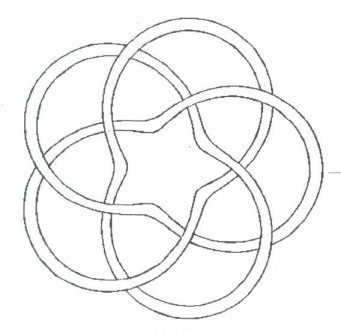Figure 12 A (5,3) Torus Knot This uses an Additive Model, and it differs from a Turks-Head Knot in that the weave is Not alternating. R = ( 1.75, 2.00 ) + cos( 5q/ 3 ) ( 0 < q < 1080 )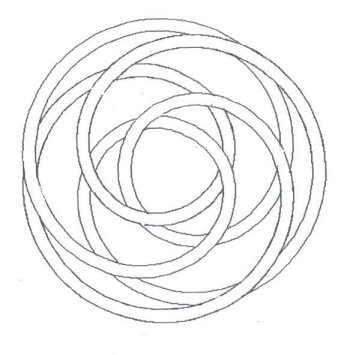Figure 13 A (3,5) Torus Knot. This may be changed to and from the (5,3) Torus Knot shown in Figure 12. This is also an additive model. R = ( 1.75, 2.00 ) + cos( 3q/ 5 ) ( 0 < q < 1800 )Figure 14 A (4,3) Torus Knot. This utilizes a Power Curve. R = ( 0.25, 0.50 ) + 2 cos( 4q/ 3 )Figure 15 A (3,4) Torus Knot, Which may change to and from the (4,3) Torus Knot shown in Figure 14. R = ( 0.25, 0.50 ) + 2cos( 3q/ 4 ) ( 0 < q < 4 × 360 = 1440 )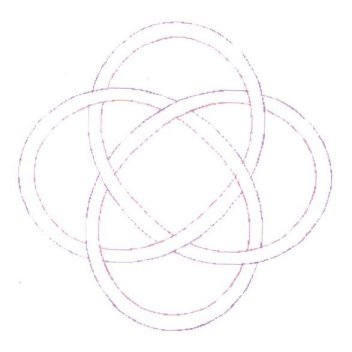Figure 16 A 4-Bight, 3-Lead Turks-Head Knot. This has an Alternating weave, but its underlying graph is the same as the underlying graph for Figure 14, which shows a Torus Knot. It also uses a power curve. Figures 17-20 demonstrate a set of possibilities which arise from using the polar trig model, a 2B, 3L Power Curve. Which brings us back full circle, to the beginning.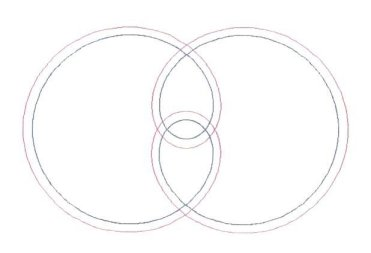Figure 17 A Basic Underlying Design/Graph. R = ( 0.00, 0.25 ) + 4cos(2q/ 3 ) ( 0 < q < 3 × 360 = 1080 )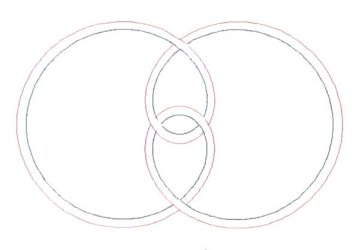Figure 18 It is also a 2-Bight, 3_Lead Turks-Head Knot, drawn from the basic underlying dessign and equations shown in Figure 17. It has an Alternating structure.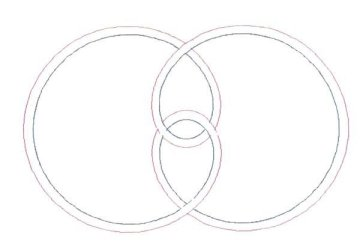Figure 19 A (2,3) Torus Knot This is also drawn from the basic design and equations shown above in Figure 17. It has a non-alternating structure. It may be changed into the next knot.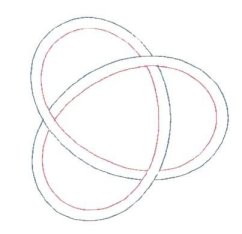Figure 20 This is one of two possible 3-Bight, 2-Lead Turks-Head Knots, and is one of two (3,2) Torus Knots at the same time. Which brings us back full circle to the beginning. R = ( 0.6, 0.9 ) + 2cos( 3q/ 2) However, before finishing this article, the author must restate the old adage that Knots are a book which has no end, and leave off with a couple of personal artistic designs in Figures 21-22.Figure 21 A Dove Silhouette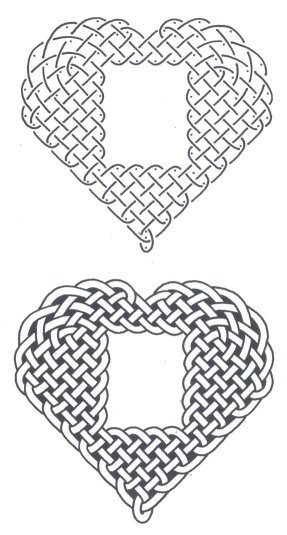Figure 22 A Heart Frame * * * The author would like to thank Professor Slavik Jablan for all he has done in converting the paper graphs to electronic format, and for composing the material. The author would also like to thank Rosalyn R. Carpenter and Thomas M. Hughes for their assistance in proofreading and readibility of this article. The author would like to thank Professor Ann Wagner of Towson University for her encouragement and suggestions regarding polar coordinate graphing. Aside from searching the Internet, to no avail, for graphs similar to the ones presented in this article, the author would like to cite the following two texts as sources: Adams, Colin C., The KNOT Book, An Elementary Introduction to the Mathematical Theory of Knots, W.H. Freeman and Company, New York, New York, 2001. This book was given to the articles author by Phyllis J. Robinson. Ashley, Clifford W., The Ashley Book of Knots, Doubleday & Company, Inc., Garden City, New York, 1944. A copy of this book was given to the author by Wallace McCurdy and Family, including Martha. Skip Pennock, Member, The International Guild of Knot Tyers (The IGKT) The IGKT Website: www.igkt.net Thursday, 19 August, 2004